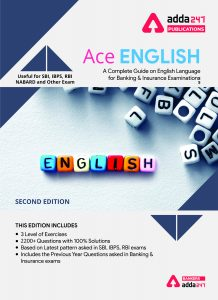Latest Banking jobs   »   English Quizzes for SBI Clerk Mains...

# English Quizzes for SBI Clerk Mains 2021 – 11th August

Directions (1-5): A number of sentences are given below which, when properly sequenced, form a COHERENT PARAGRAPH. Choose the most LOGICAL ORDER of sentence from the choices given to construct a COHERENT PARAGRAPH.

(A) It reverberates throughout the entire Universe. And you are transmitting that frequency with your thoughts!
(B) The frequency you transmit reaches beyond cities, beyond countries, beyond the world.
(C) You are a human transmission tower, and you are
(D) More powerful than any television tower created on earth.

Q1. Which of the following will be the SECOND sentence after rearrangement?
(a) A
(b) B
(c) C
(d) D
(e) E

Q2. Which of the following will be the FIRST sentence after rearrangement?
(a) A
(b) B
(c) C
(d) D
(e) E

Q3. Which of the following will be the FOURTH sentence after rearrangement?
(a) A
(b) B
(c) C
(d) D
(e) E

Q4. Which of the following will be the THIRD sentence after rearrangement?
(a) A
(b) B
(c) C
(d) D
(e) E

Q5. Which of the following will be the LAST(FIFTH) sentence after rearrangement?
(a) A
(b) B
(c) C
(d) D
(e) E

Directions (6-10): A number of sentences are given below which, when properly sequenced, form a COHERENT PARAGRAPH. Choose the most LOGICAL ORDER of sentence from the choices given to construct a COHERENT PARAGRAPH.
(A) Asian economies will need alternative sources of growth to compensate for the rapid fall in demand from the western markets.
(B) But the crisis has exposed the limits of region’s dominant economic-growth model.
(C) The export-led model that propelled many Asian economies so effectively for the past 30 years must be adapted to a different global economic context.
(D) Asian is less exposed to the financial turmoil than the west is, because Asian countries responded to the previous decade’s regional crisis by improving
(E) Their current-account positions, accumulating reserves, and ensuring that their banking systems operated prudently.

Q6. Which of the following will be the FIRST sentence after rearrangement?
(a) A
(b) B
(c) C
(d) D
(e) E

Q7. Which of the following will be the SECOND sentence after rearrangement?
(a) A
(b) B
(c) C
(d) D
(e) E

Q8. Which of the following will be the LAST(FIFTH) sentence after rearrangement?
(a) A
(b) B
(c) C
(d) D
(e) E

Q9. Which of the following will be the FOURTH sentence after rearrangement?
(a) A
(b) B
(c) C
(d) D
(e) E

Q10. Which of the following will be the THIRD sentence after rearrangement?
(a) A
(b) B
(c) C
(d) D
(e) E

Directions (11-15): A number of sentences are given below which, when properly sequenced, form a COHERENT PARAGRAPH. Choose the most LOGICAL ORDER of sentence from the choices given to construct a COHERENT PARAGRAPH.
(A) The dangers of conflicting irrational majoritarianism with enlightened consensus are, indeed, great in developing democracy.
(B) Real democracy is about mediating the popular will through a network of institutional structure and the law of the land.
(C) While law making and governance are meant to articulate the latter, the judiciary is supposed to protect the former from any kind of excess that might occur,
(D) Unwittingly or otherwise, in the conduct of legislative and governmental functions.
(E) The principle of separation of powers is meant to embody a desirable tension between individual rights and social consensus.

Q11. Which of the following will be the LAST(FIFTH) sentence after rearrangement?
(a) A
(b) B
(c) C
(d) D
(e) E

Q12. Which of the following will be the FIRST sentence after rearrangement?
(a) A
(b) B
(c) C
(d) D
(e) E

Q13. Which of the following will be the SECOND sentence after rearrangement?
(a) A
(b) B
(c) C
(d) D
(e) E

Q14. Which of the following will be the THIRD sentence after rearrangement?
(a) A
(b) B
(c) C
(d) D
(e) E

Q15. Which of the following will be the FOURTH sentence after rearrangement?
(a) A
(b) B
(c) C
(d) D
(e) E

Solutions

S1. Ans.(d)
Sol.  CDEBA; D

S2. Ans.(c)
Sol. C

S3. Ans.(b)
Sol. B

S4. Ans.(e)
Sol. E

S5. Ans.(a)
Sol. A

S6. Ans.(d)
Sol. DEBAC; D

S7. Ans.(e)
Sol. E

S8. Ans.(c)
Sol. C

S9. Ans.(a)
Sol. A

S10. Ans.(b)
Sol. B

S11. Ans.(b)
Sol. ECDAB; B

S12. Ans.(e)
Sol. E

S13. Ans.(c)
Sol. C

S14. Ans.(d)
Sol. D

S15. Ans.(a)
Sol. A